# CBSE Question Paper 2010 class 12 Chemistry## myCBSEguide App

CBSE, NCERT, JEE Main, NEET-UG, NDA, Exam Papers, Question Bank, NCERT Solutions, Exemplars, Revision Notes, Free Videos, MCQ Tests & more.

CBSE Question Paper 2010 class 12 Chemistry conducted by Central Board of Secondary Education, New Delhi in the month of March 2010. CBSE previous year question papers with the solution are available in the myCBSEguide mobile app and website. The Best CBSE App for students and teachers is myCBSEguide which provides complete study material and practice papers to CBSE schools in India and abroad.

CBSE Question Paper 2010 class 12 Chemistry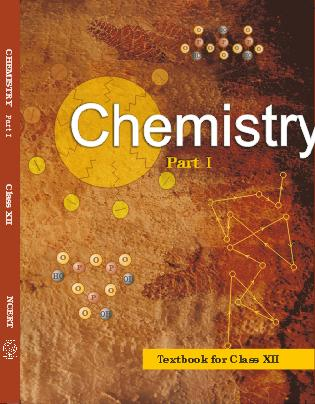## Class 12 Chemistry list of chapters

1. The Solid State
2. Solutions
3. Electrochemistry
4. Chemical Kinetics
5. Surface Chemistry
6. General Principles and Processes of Isolation of Elements
7. The p-Block Elements
8. The d and f Block Elements
9. Coordination Compounds
10. Haloalkanes and Haloarenes
11. Alcohols, Phenols and Ethers
12. Aldehydes, Ketones and Carboxylic Acids
13. Amines
14. Biomolecules
15. Polymers
16. Chemistry in Everyday life

## CBSE Question Paper 2010 class 12 Chemistry

### General Instructions

1. All questions are compulsory.
2. Marks for each question are indicated against it.
3. Question numbers 1 to 8 are very short-answer questions, carrying 1 mark each. Answer these in one word or about one sentence each.
4. Question numbers 9 to 18 are short-answer questions, carrying 2 marks each. Answer these in about 30 words each.
5. Question numbers 19 to 27 are short-answer questions of 3 marks each. Answer these in about 40 words each.
6. Question numbers 28 to 30 are long-answer questions of 5 marks each. Answer these in about 70 words each.
7. Use Log Tables, if necessary Use of calculators is not permitted.

1. Write a feature which will distinguish a metallic solid from an ionic solid. 

2. Define ‘order of a reaction’. 

3. What is an emulsion? 

4. Why does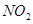dimerise? 

5. Give an example of linkage isomerism. 

6. A solution of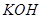hydrolyses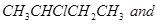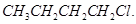Which one of these is more easily hydrolysed? 

7. Draw the structural formula of I-phenylpropan-l-one molecule. 

8. Give the IUPAC name of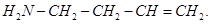9. Non-ideal solutions exhibit either positive or negative deviations from Raoult’s law. What are these deviations and why are they caused? Explain with one example for each type. 

10. A reaction is of first order in reactant A and of second order in reactant B. How is the rate of this reaction affected when (i) the concentration of B alone is increased to three times (ii) the concentrations of A as well as B are doubled? 

11. The rate constant for a reaction of zero order in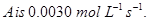How long will it take for the initial concentration of A to fall from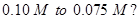12. Draw the structures of white phosphorus and red phosphorus. Which one of these two types of phosphorus is more reactive and why? 

13. Explain the following observations:

(i) Generally, there is an increase in density of elements from titanium (Z = 22) to copper (Z = 29) in the first series of transition elements.

(ii) Transition elements and their compounds are generally found to be good catalysts in chemical reactions. 

14. Name the following coordination compounds according to IUPAC system of nomenclature: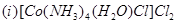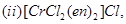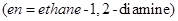15. Illustrate the following reactions giving a chemical equation for each:

(i) Kolbe’s reaction,

(ii) Williamson synthesis. 

16. How are the following conversions carried out?

(i) Benzyl chloride to benzyl alcohol,

(ii) Methyl magnesium bromide to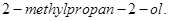17. Explain the following terms:

(i) Invert sugar

(ii) Polypeptides

OR

Name the products of hydrolysis of sucrose. Why is sucrose not a reducing sugar? 

18. What are essential and non-essential amino acids in human food? Give one example of each type. 

19. The well-known mineral fluorite is chemically calcium fluoride. It is known that in one unit cell of this mineral there are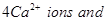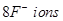and that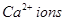are arranged in a fcc lattice. The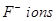fill all the tetrahedral holes in the face centred cubic lattice of. The edge of the unit cell is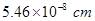in length. The density of the solid is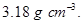Use this information to calculate Avogadro’s number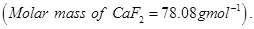20. A solution prepared by dissolving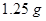of oil of winter green (methyl salicylate) in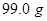of benzene has a boiling point of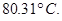Determine the molar mass of this compound.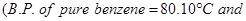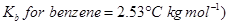21. What is the difference between multi molecular and macromolecular colloids? Give one example of each type. How are associated colloids different from these two types of colloids? 

22. Describe how the following changes are brought about:

(i) Pig iron into steel.

(ii) Zinc oxide into metallic zinc.

(iii) Impure titanium into pure titanium. 

OR

Describe the role of

(i)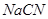in the extraction of gold from gold are.

(ii)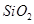in the extraction of copper from copper matte.

(iii) Iodine in the refining of zirconium.

Write chemical equations for the involved reactions.

23. How would you account for the following?

(i) The atomic radii of the metals of the third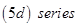of transition elements are virtually the same as those of the corresponding members of the second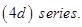(ii) The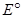value for the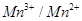couple is much more positive than that for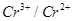couple or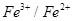couple.

(iii) The highest oxidation state of a metal is exhibited in its oxide or fluoride. 

24. (i) State one use each of DDT and iodoform.

(ii) Which compound in the following couples will react faster in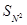displacement and why?

(a) 1-Bromopentane or 2-bromopentane

(b) 1-Bromo-2-methylbutane or 2-bromo-2-methylbutane. 

25. In the following cases rearrange the compounds as directed: 

(i) In an increasing order of basic strength: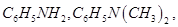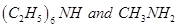(ii) In a decreasing order of basic strength:

Aniline, p-nitroaniline and p-toluidine

(iii) In an increasing order of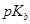values: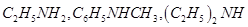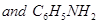26. Give one example each of

(ii) condensation polymers,

(iii) copolymers. 

27. What are analgesic medicines? How are they classified and when are they commonly recommended for use? 

28. (a) State Kohlrausch law of independent migration of ions. Write an expression for the molar conductivity of acetic acid at infinite dilution according to Kohlrausch law.

(b) Calculate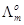for acetic acid.

Given that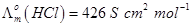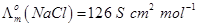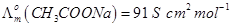OR

(a) Write the anode and cathode reactions and the overall reaction occurring in a lead storage battery.

(b) A copper-silver cell is set up. The copper ion concentration is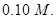The concentration of silver ion is not known. The cell potential when measured was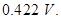Determine the concentration of silver ions in the cell.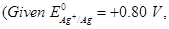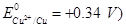29. (a) Complete the following chemical equations: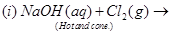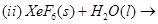(b) How would you account for the following?

(i) The value of electron gain enthalpy with negative sign for sulphur is higher than that for oxygen.

(ii)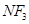is an exothermic compound but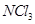is endothermic compound.

(iii)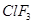molecule has a T-shaped structure and not a trigonal planar one. 

OR

(a) Complete the following chemical reaction equations: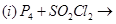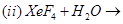(b) Explain the following observations giving appropriate reasons:

(i) The stability of +5 oxidation state decreases down the group in group 15 of the periodic table.

(ii) Solid phosphorus pentachloride behaves as an ionic compound.

(iii) Halogens are strong oxidizing agents. 

30. (a) Explain the mechanism of a nucleophilic attack on the carbonyl group of an alciehyde or a ketone.

(b) An organic compound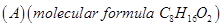was hydrolysed with dilute sulphuric acid to give a carboxylic acid (B) and an alcohol (C). Oxidation of (C) with chromic acid also produced (B). On dehydration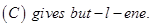Write the equations for the reactions involved. 

OR

(a) Give chemical tests to distinguish between the following pairs of compounds:

(i) Ethanal and Propanal

(ii) Phenol and Benzoic acid

(b) How will you bring about the following conversions? 5

(i) Benzoic acid to benzaldehyde

(ii) Ethanal to but-2-enal

(iii) Propanone to propane

Give complete reaction in each case.

These are questions only. To view and download complete question paper with solution install myCBSEguide App from google play store or login to our student dashboard.

## Chemistry Question Paper 2010

Download class 12 Chemistry question paper with solution from best CBSE App the myCBSEguide. CBSE class 12 Chemistry question paper 2010 in PDF format with solution will help you to understand the latest question paper pattern and marking scheme of the CBSE board examination. You will get to know the difficulty level of the question paper. CBSE question papers 2010 for class 12 Chemistry have 30 questions with solution.

## Previous Year Question Paper for class 12 in PDF

CBSE question papers 2018, 2017, 2016, 2015, 2014, 2013, 2012, 2011, 2010, 209, 2008, 2007, 2006, 2005 and so on for all the subjects are available under this download link. Practicing real question paper certainly helps students to get confidence and improve performance in weak areas.

To download CBSE Question Paper 2010 class 12 Accountancy, Chemistry, Physics, History, Political Science, Economics, Geography, Computer Science, Home Science, Business Studies and Home Science; do check myCBSEguide app or website. myCBSEguide provides sample papers with solution, test papers for chapter-wise practice, NCERT solutions, NCERT Exemplar solutions, quick revision notes for ready reference, CBSE guess papers and CBSE important question papers. Sample Paper all are made available through the best app for CBSE students and myCBSEguide website.